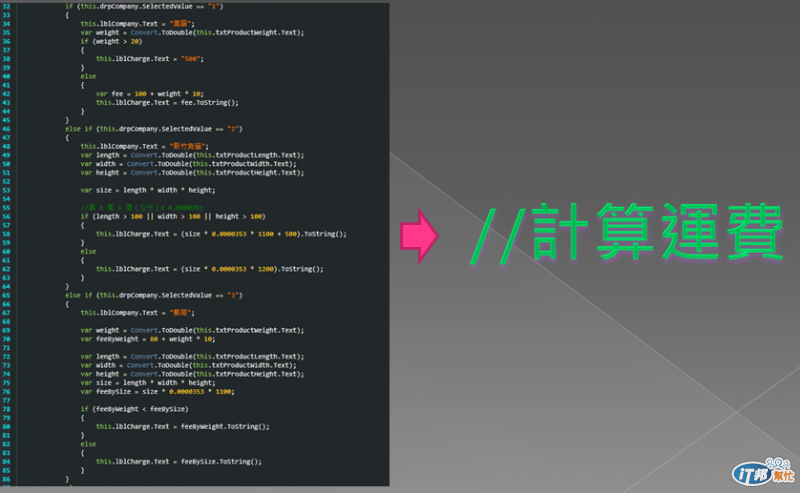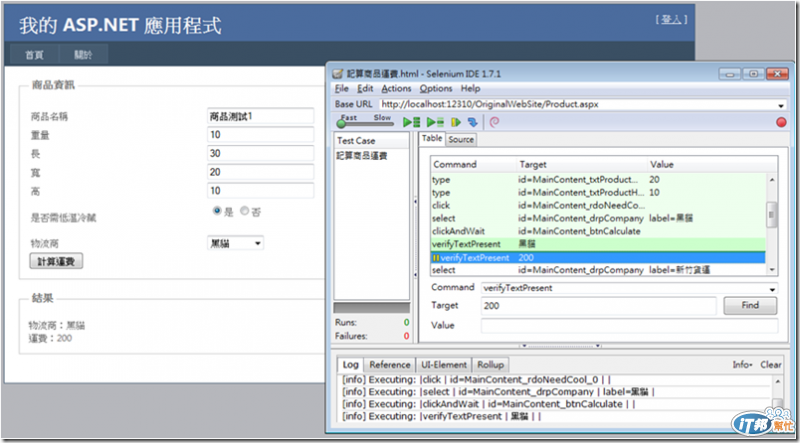### [30天快速上手TDD][Day 11]Refactoring - 讓程式碼說話

[30天快速上手TDD][Day 11]Refactoring - 讓程式碼說話

### 目前的程式碼


protected void btnCalculate_Click(object sender, EventArgs e)
{
if (this.IsValid)
{
if (this.drpCompany.SelectedValue == "1")
{
this.lblCompany.Text = "黑貓";
var weight = Convert.ToDouble(this.txtProductWeight.Text);
if (weight > 20)
{
this.lblCharge.Text = "500";
}
else
{
var fee = 100 + weight * 10;
this.lblCharge.Text = fee.ToString();
}
}
else if (this.drpCompany.SelectedValue == "2")
{
this.lblCompany.Text = "新竹貨運";
var length = Convert.ToDouble(this.txtProductLength.Text);
var width = Convert.ToDouble(this.txtProductWidth.Text);
var height = Convert.ToDouble(this.txtProductHeight.Text);

var size = length * width * height;

//長 x 寬 x 高（公分）x 0.0000353
if (length > 100 || width > 100 || height > 100)
{
this.lblCharge.Text = (size * 0.0000353 * 1100 + 500).ToString();
}
else
{
this.lblCharge.Text = (size * 0.0000353 * 1200).ToString();
}
}
else if (this.drpCompany.SelectedValue == "3")
{
this.lblCompany.Text = "郵局";

var weight = Convert.ToDouble(this.txtProductWeight.Text);
var feeByWeight = 80 + weight * 10;

var length = Convert.ToDouble(this.txtProductLength.Text);
var width = Convert.ToDouble(this.txtProductWidth.Text);
var height = Convert.ToDouble(this.txtProductHeight.Text);
var size = length * width * height;
var feeBySize = size * 0.0000353 * 1100;

if (feeByWeight < feeBySize)
{
this.lblCharge.Text = feeByWeight.ToString();
}
else
{
this.lblCharge.Text = feeBySize.ToString();
}
}
else
{
var js = "alert('發生不預期錯誤，請洽系統管理者');location.href='http://tw.yahoo.com/';";
this.ClientScript.RegisterStartupScript(this.GetType(), "back", js, true);
}
}
}

### 重構第二式：說人話protected void btnCalculate_Click(object sender, EventArgs e)
{
if (this.IsValid)
{
//選黑貓，計算出運費，呈現物流商名稱與運費
if (this.drpCompany.SelectedValue == "1")
{
this.lblCompany.Text = "黑貓";
var weight = Convert.ToDouble(this.txtProductWeight.Text);
if (weight > 20)
{
this.lblCharge.Text = "500";
}
else
{
var fee = 100 + weight * 10;
this.lblCharge.Text = fee.ToString();
}
}
//選新竹貨運，計算出運費，呈現物流商名稱與運費
else if (this.drpCompany.SelectedValue == "2")
{
this.lblCompany.Text = "新竹貨運";
var length = Convert.ToDouble(this.txtProductLength.Text);
var width = Convert.ToDouble(this.txtProductWidth.Text);
var height = Convert.ToDouble(this.txtProductHeight.Text);

var size = length * width * height;

//長 x 寬 x 高（公分）x 0.0000353
if (length > 100 || width > 100 || height > 100)
{
this.lblCharge.Text = (size * 0.0000353 * 1100 + 500).ToString();
}
else
{
this.lblCharge.Text = (size * 0.0000353 * 1200).ToString();
}
}
//選郵局，計算出運費，呈現物流商名稱與運費
else if (this.drpCompany.SelectedValue == "3")
{
this.lblCompany.Text = "郵局";

var weight = Convert.ToDouble(this.txtProductWeight.Text);
var feeByWeight = 80 + weight * 10;

var length = Convert.ToDouble(this.txtProductLength.Text);
var width = Convert.ToDouble(this.txtProductWidth.Text);
var height = Convert.ToDouble(this.txtProductHeight.Text);
var size = length * width * height;
var feeBySize = size * 0.0000353 * 1100;

if (feeByWeight < feeBySize)
{
this.lblCharge.Text = feeByWeight.ToString();
}
else
{
this.lblCharge.Text = feeBySize.ToString();
}
}
//發生預期以外的狀況，呈現警告訊息，回首頁
else
{
var js = "alert('發生不預期錯誤，請洽系統管理者');location.href='http://tw.yahoo.com/';";
this.ClientScript.RegisterStartupScript(this.GetType(), "back", js, true);
}
}
}

1. 選了哪一間物流商。
2. 計算出運費。
3. 呈現物流商的名稱與運費結果。
4. 防呆的處理。

### 重構第三式：垃圾分類

1. 把原本人話所描述的區塊選取後；
2. 按滑鼠右鍵，選擇「重構」；
3. 選擇「擷取方法」

### 垃圾分類完的版本


protected void btnCalculate_Click(object sender, EventArgs e)
{
//若頁面通過驗證
if (this.IsValid)
{
//選黑貓，計算出運費，呈現物流商名稱與運費
if (this.drpCompany.SelectedValue == "1")
{
CalculatedByBlackCat();
}
//選新竹貨運，計算出運費，呈現物流商名稱與運費
else if (this.drpCompany.SelectedValue == "2")
{
CalculatedByHsinchu();
}
//選郵局，計算出運費，呈現物流商名稱與運費
else if (this.drpCompany.SelectedValue == "3")
{
CalculatedByPostOffice();
}
//發生預期以外的狀況，呈現警告訊息，回首頁
else
{
var js = "alert('發生不預期錯誤，請洽系統管理者');location.href='http://tw.yahoo.com/';";
this.ClientScript.RegisterStartupScript(this.GetType(), "back", js, true);
}
}
}

private void CalculatedByPostOffice()
{
this.lblCompany.Text = "郵局";

var weight = Convert.ToDouble(this.txtProductWeight.Text);
var feeByWeight = 80 + weight * 10;

var length = Convert.ToDouble(this.txtProductLength.Text);
var width = Convert.ToDouble(this.txtProductWidth.Text);
var height = Convert.ToDouble(this.txtProductHeight.Text);
var size = length * width * height;
var feeBySize = size * 0.0000353 * 1100;

if (feeByWeight < feeBySize)
{
this.lblCharge.Text = feeByWeight.ToString();
}
else
{
this.lblCharge.Text = feeBySize.ToString();
}
}

private void CalculatedByHsinchu()
{
this.lblCompany.Text = "新竹貨運";
var length = Convert.ToDouble(this.txtProductLength.Text);
var width = Convert.ToDouble(this.txtProductWidth.Text);
var height = Convert.ToDouble(this.txtProductHeight.Text);

var size = length * width * height;

//長 x 寬 x 高（公分）x 0.0000353
if (length > 100 || width > 100 || height > 100)
{
this.lblCharge.Text = (size * 0.0000353 * 1100 + 500).ToString();
}
else
{
this.lblCharge.Text = (size * 0.0000353 * 1200).ToString();
}
}

private void CalculatedByBlackCat()
{
this.lblCompany.Text = "黑貓";
var weight = Convert.ToDouble(this.txtProductWeight.Text);
if (weight > 20)
{
this.lblCharge.Text = "500";
}
else
{
var fee = 100 + weight * 10;
this.lblCharge.Text = fee.ToString();
}
}### 小結

1. 若頁面通過驗證，則
2. 當選[黑貓]時，計算出運費，呈現物流商名稱與運費；
3. 當選[新竹貨運]時，計算出運費，呈現物流商名稱與運費；
4. 當選[郵局]時，計算出運費，呈現物流商名稱與運費；
5. 當發生預期以外的狀況時，呈現警告訊息，回首頁。想收到第一手公開培訓課程資訊，或想詢問企業內訓、顧問、教練、諮詢服務的，請洽 Facebook 粉絲專頁：91敏捷開發之路。